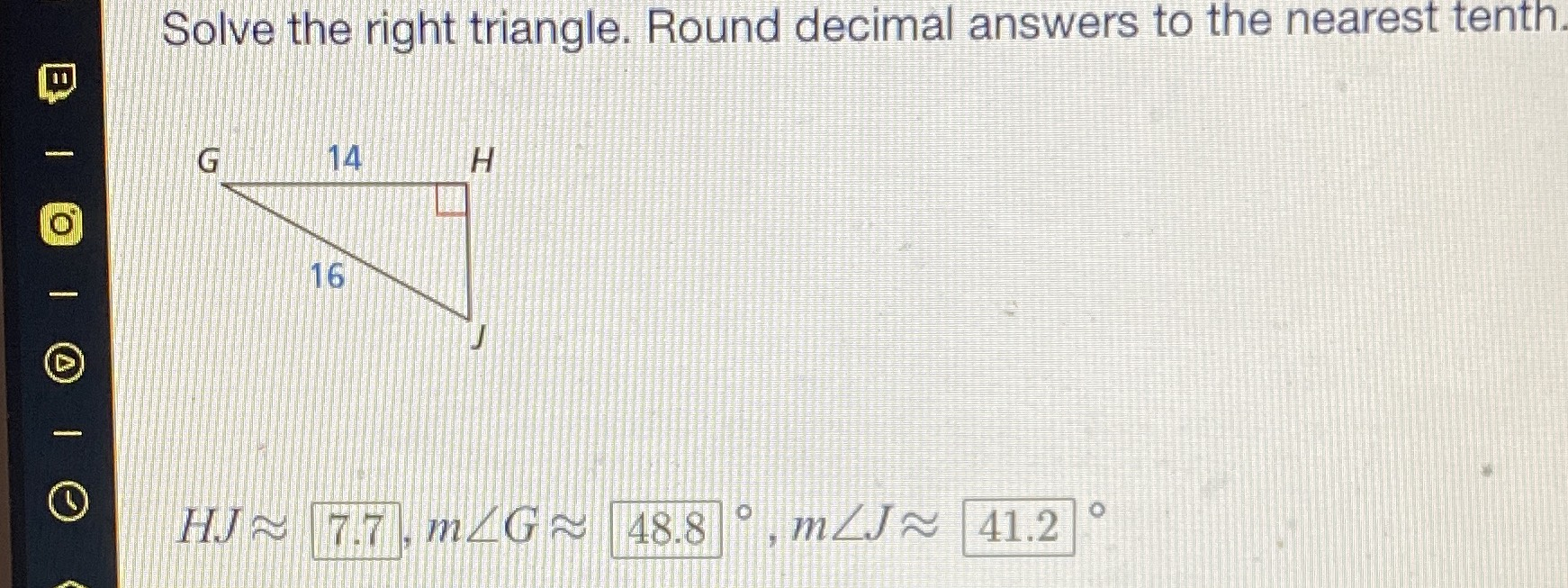### Still have math questions?

Trigonometry
QuestionSolve the right triangle. Round decimal answers to the nearest tenth.

$$28.955°$$
$$61.045°$$